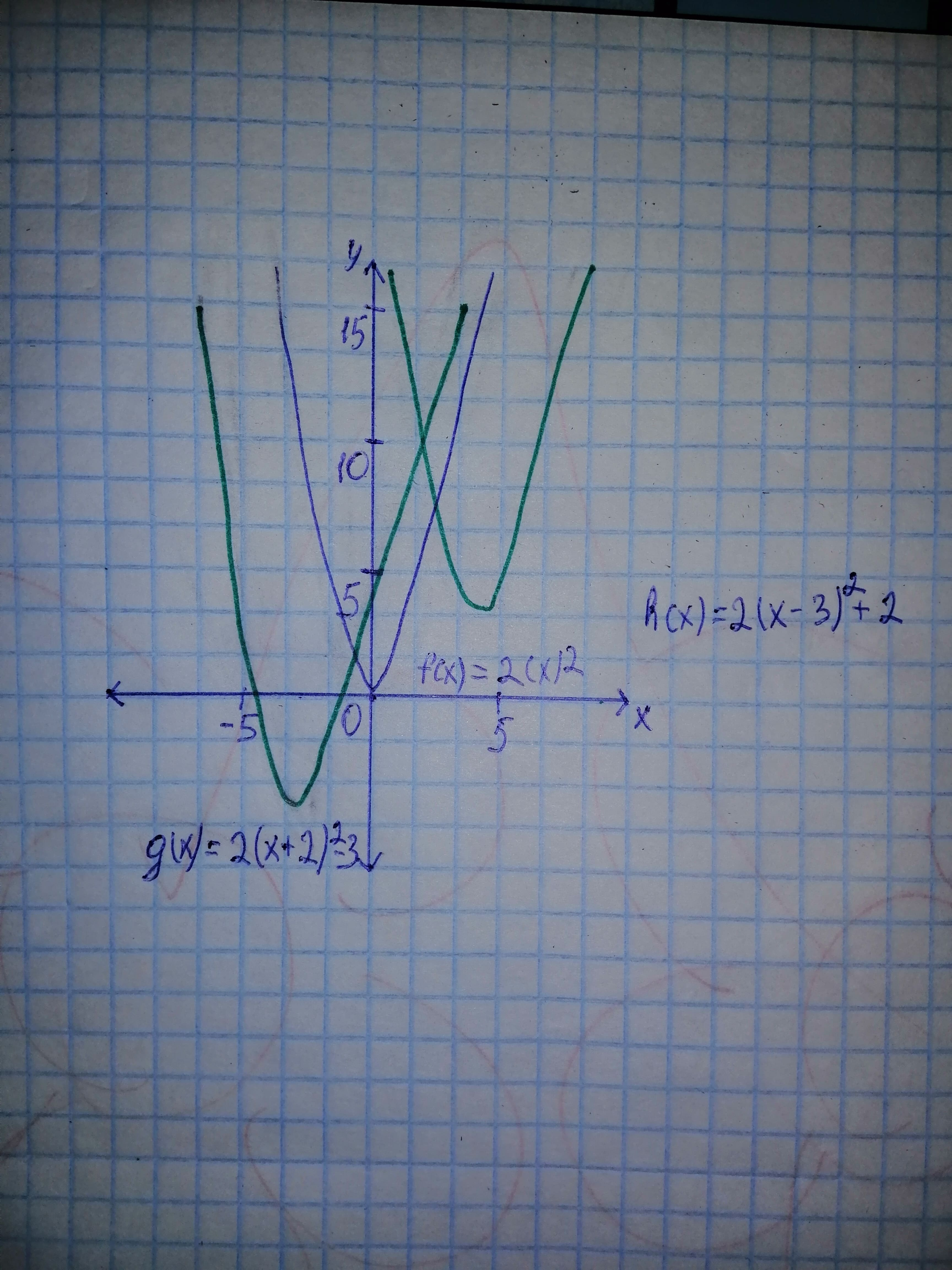# Describe how to obtain the graph of g and f if g(x)=2(x + 2)^{2} - 3, h(x)=2x^{2}, and h(x)=2(x - 3)^{2} + 2aflacatn 2021-03-08 Answered
Describe how to obtain the graph of g and f if
You can still ask an expert for help

• Questions are typically answered in as fast as 30 minutes

Solve your problem for the price of one coffee

• Math expert for every subject
• Pay only if we can solve itLayton

Step 1
Properties of the transformation of the graph:
let $y=f\left(x\right)=2{x}^{2}.$
1) shift right by a unit.
2) shift left by a unit.
3) shift upward by b units.
4) shift downward by b units.
5) graph stretched expand vertically if
and compressed vertically if

Step 2
a) For graph
Translate $f\left(x\right)=2{x}^{2}$ by 2 units left and expand vertically by 2 units then shift it down by 3 units you get

b) For graph
Translate $f\left(x\right)=2{x}^{2}$ by 3 units left and expand vertically by 2 units then shift it down by 2 units you get

The graph of all functions are shown in below: